Courses

JEE Main Previous year questions (2016-20): Electromagnetic Induction & Alternating Current- 2 Notes | Study Physics For JEE - JEE

JEE: JEE Main Previous year questions (2016-20): Electromagnetic Induction & Alternating Current- 2 Notes | Study Physics For JEE - JEE

The document JEE Main Previous year questions (2016-20): Electromagnetic Induction & Alternating Current- 2 Notes | Study Physics For JEE - JEE is a part of the JEE Course Physics For JEE.
All you need of JEE at this link: JEE

Q 34. An ideal capacitor of capacitance 0.2 µF is charged to a potential difference of 10 V. The charging battery is then disconnected. The capacitor is then connected to an ideal inductor of self-inductance 0.5 mH. The current at a time when the potential difference across the capacitor is 5 V, is:     
(1) 0.34 A
(2) 0.25 A
(3) 0.15 A
(4) 0.17 A
Ans:
(4)
Solution:

Qo = 0.2 × 10 µC = 2µC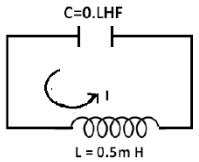Vo = 10V
Ei of capacitor = 1/2 × 0.2 µf × (10V)2
= 10µJ
Ef of capacitor = 1/2 × 0.2µf × (5V)2
= 2.5 µj
Einductor  = 7.5 µJ = 1/2 Li2
⇒ 7.5 × 10−6 = 1/2 × 0.5 × 10−3 × i2
⇒ 30 × 10−3 = i2
⇒ i = √3/10 = 0.17 A

Q 35.  A power transmission line feeds input power at 2300V to a step down transformer with its primary windings having 4000 turns giving the output power at 230V. If the current in the primary coil of the transformer is 5A and its efficiency is 90% the output current would be:    
(1) 45A
(2) 50A
(3) 20A
(4) 25A
Ans:
A
Solution:

We know, formula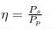where, η is efficiency, Px denotes pawar of secondary transmission, Pη
denotes power of primary transmission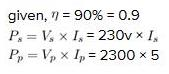so, 0.9 = 230 x Ix / 2300 x 5
hence, Ix = 45A
therefore, output current would be 45A

Q 36.  A plane electromagnetic wave of wavelength λ has an intensity I. It is propagating along the positive Y-direction. The allowed expressions for the electric and magnetic fields are given by:    
(1)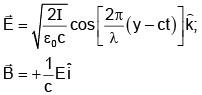(2)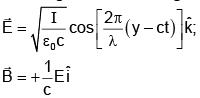(3)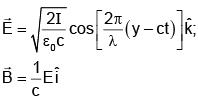(4)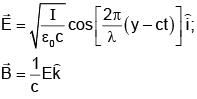Ans:
A
Solution:
If E0 is magnitude of electric field then 1/2ε0E2 x C = I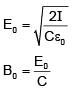direction of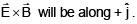Q 37. A charge q is spread uniformly over an insulated loop of radius r. If it is rotated with an angular velocity ω with respect to normal axis then magnetic moment of the loop is:    
(1)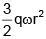(2)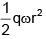(3) qωr2
(4)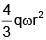Ans:
(2)
Solution: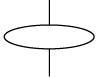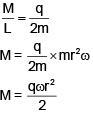Q 38. A coil of cross-sectional area A having N turns is placed in a uniform magnetic field B. When it is rotated with an angular velocity ω, the maximum e.m.f. induced in the coil will be:    
(1) 3/2NBAω
(2) 3NBAω
(3) NBAω
(4) 1/2NBAω
Ans:
(3)
Solution:
Given, a coil of N turns having an area A, rotated with angular speed 'ω' in a uniform magnetic field 'B' connected to a resistor ' R'.
The flux linking to coil, φ = NBA sin (ωt)
Therefore,
Induced EMF,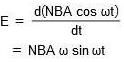When, sinωt = ±1, the induced emf value is maximum.
∴ the maximum EMF, E0 = NBAω

Q 39. In a coil of resistance 100 Ω, a current is induced by changing the magnetic flux through it as shown in the figure. The magnitude of change in flux through the coil is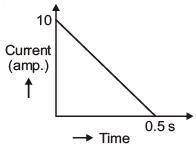(1) 250 Wb
(2) 275 Wb
(3) 200 Wb
(4) 225 Wb
Ans
: (1)
Solution: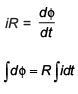Magnitude of change in flux = R × area under current vs time graph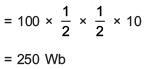Q 40. A small circular loop of wire of radius a is located at the centre of a much larger circular wire loop of radius b. The two loops are in the same plane. The outer loop of radius b carries an alternating current I = I0 cos (ωt). The emf induced in the smaller inner loop is nearly ?    
(1)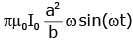(2)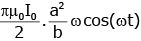(3)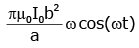(4)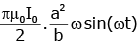Ans:
(4)
Solution:
Magnetic field due to outer current loop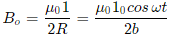Induced emf in inner loop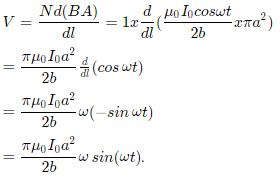Q 41. Magnetic field in a plane electromagnetic wave is given by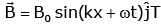Expression for corresponding electric field will be    
(1)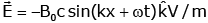(2)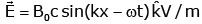(3)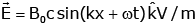(4)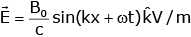Ans: (3)
Solution: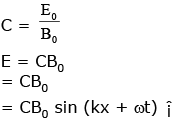Q 42. A sinusoidal voltage of peak value 283 V and angular frequency 320/s is applied to a series LCR circuit. Given that R = 5 W, L = 25 μH and C = 1000 μF. The total impedance, and phase difference between the voltage across the source and the current will respectively be -    
(1) 10 Ω and tan-1(5/3)
(2) 7 Ω and 45°
(3) 7 Ω and tan-1(5/3)
(4) 10 Ω and tan-1(8/3)
Ans:
(2)
Solution:
e0 = 283 volt    ω = 320
xL = 320 × 25 × 10-3 = 8 Ω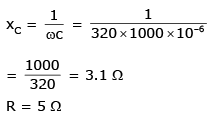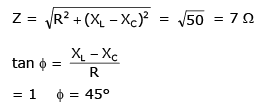Q 43. The electric field component of a monochromatic radiation is given by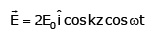Its magnetic field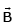is then given by: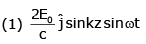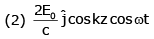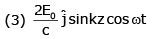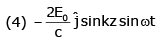Ans:
(1)
Solution: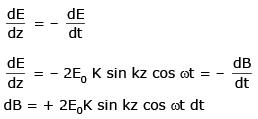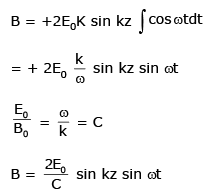Q 44. Hysteresis loops for two magnetic materials A and B are given below :
These materials are used to make magnets for electric generators, transformer core and electromagnet core.    
Then it is proper to use :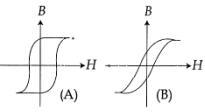(1) A for electric generators and transformers
(2) A for electromagnets and B for electric generators
(3) A for transformers and B for electric generators
(4) B for electromagnets and transformers
Ans:
(4)
Conceptual (Requires low retentivity and low coercivity)

Q 45. An arc lamp requires a direct current of 10 A at 80 V to function. It is connected to a 220 V (rms), 50 Hz AC supply, the series inductor needed for it to work is close to:    
(1) 80 H
(2) 0.08 H
(3) 0.044 H
(4) 0.065 H
Ans:
(4)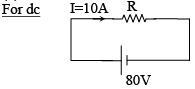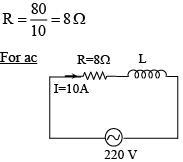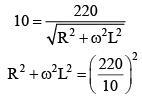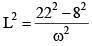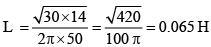Q 46. Arrange the following electromagnetic radiations per quantum in the order of increasing energy :    
A: Blue light
B: Yellow light
C: X-ray
(1) D, B, A, C
(2) A, B, D, C
(3) C, A, B, D
(4) B, A, D, C
Ans:
(1)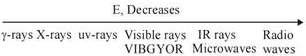(Increasing order of energy)

Q 47. A series LR circuit is connected to a voltage source with V(t) = V0 sinΩt. After very large time current I(t) behaves as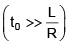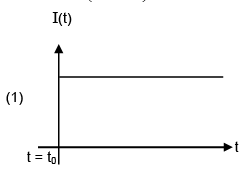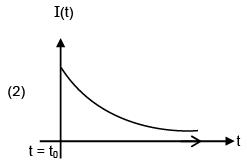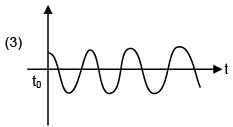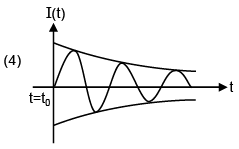Ans: (3)
Solution:
Current will be in the form of
I = I0 sin (Ωt - φ)
Graph will be sinusoidal

Q 48. The ratio (R) of output resistance r0, and the input resistance ri in measurements of input and output characteristics of a transistor is typically in the range:     
(1) R~0.1 –0.01
(2) R~0.1 –1.0
(3) R~102 –103
(4) R~1 –10
Ans:
(3)
Solution: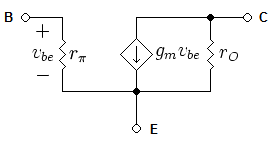The small signal model of a BJT is shown in the figure. From this figure, Rout = ro since the voltage across B and E will be 0 and hence current will only flow through r0.
Input resistance will be Rin = rπ since current will flow only through rπ
Hence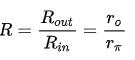Since r0 is very large and of the order of 100 kΩ and rπ  is of the order of 1- 10 kΩ, the ratio R will be of the order of 100 - 1000.

Q 49. Consider an electromagnetic wave propagating in vacuum. Choose the correct statement:
(1) For an electromagnetic wave propagating in +y direction the electric field is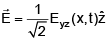and the magnetic field is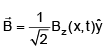(2) For an electromagnetic wave propagating in +y direction the electric field is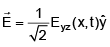and he magnetic field is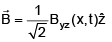(3) For an electromagnetic wave propagating in +x direction the electric field is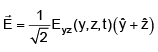and the magnetic field is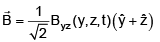(4) For an electromagnetic wave propagating in +x direction the electric field is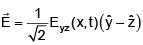and the magnetic field is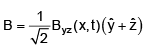Ans: (4)
Solution:
If wave is propagating in x direction,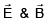must be functions of (x, t) & must be in y- z plane.

The document JEE Main Previous year questions (2016-20): Electromagnetic Induction & Alternating Current- 2 Notes | Study Physics For JEE - JEE is a part of the JEE Course Physics For JEE.
All you need of JEE at this link: JEEUse Code STAYHOME200 and get INR 200 additional OFF Use Coupon Code

Top Courses for JEE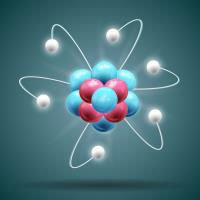Physics For JEE

187 videos|516 docs|256 tests

Top Courses for JEETrack your progress, build streaks, highlight & save important lessons and more!

,

,

,

,

,

,

,

,

,

,

,

,

,

,

,

,

,

,

,

,

,

;